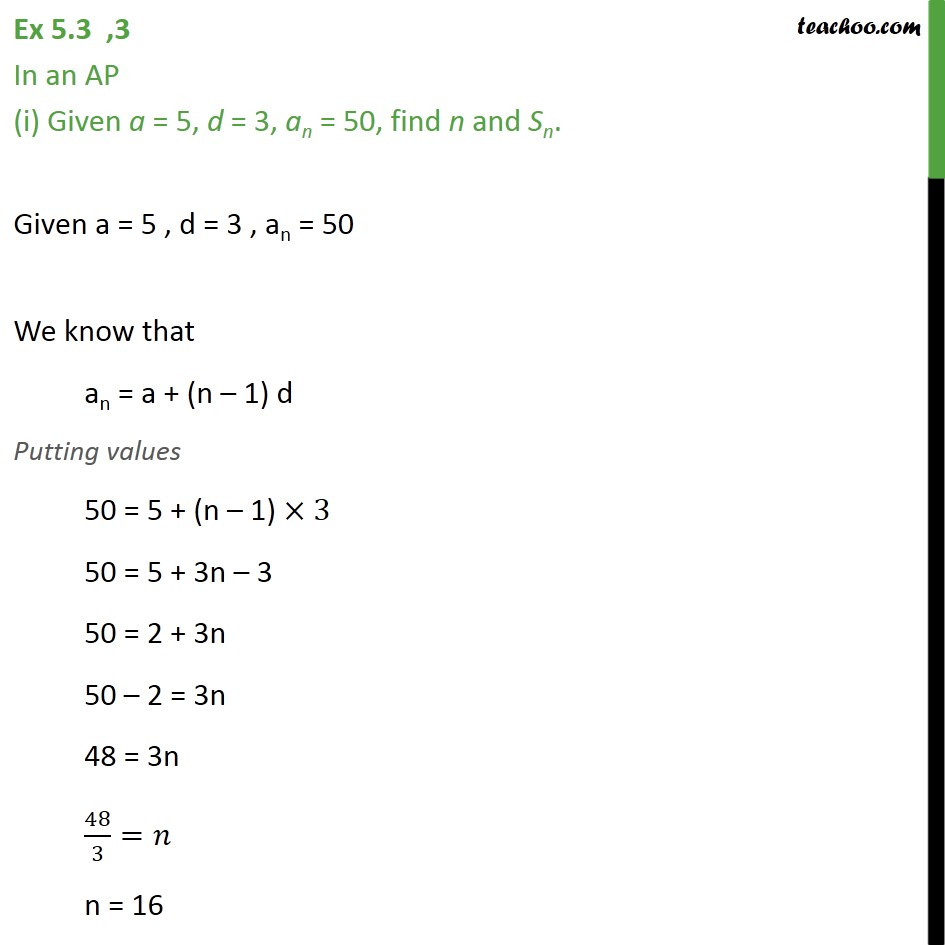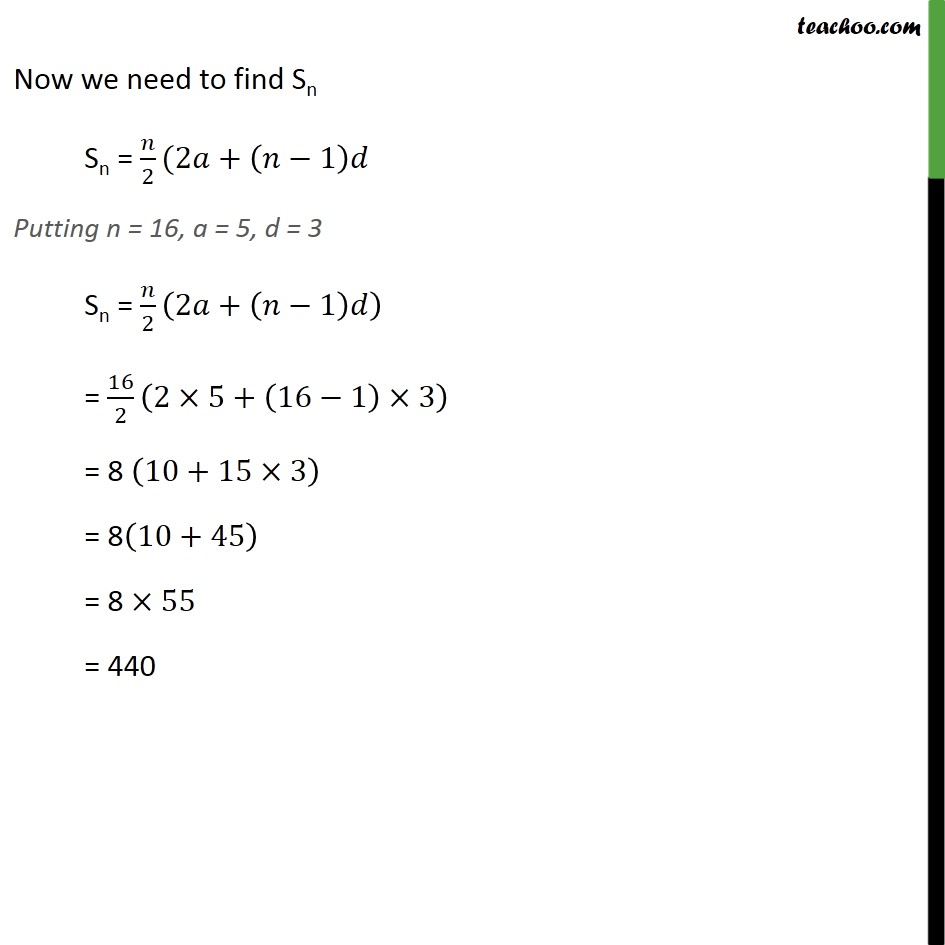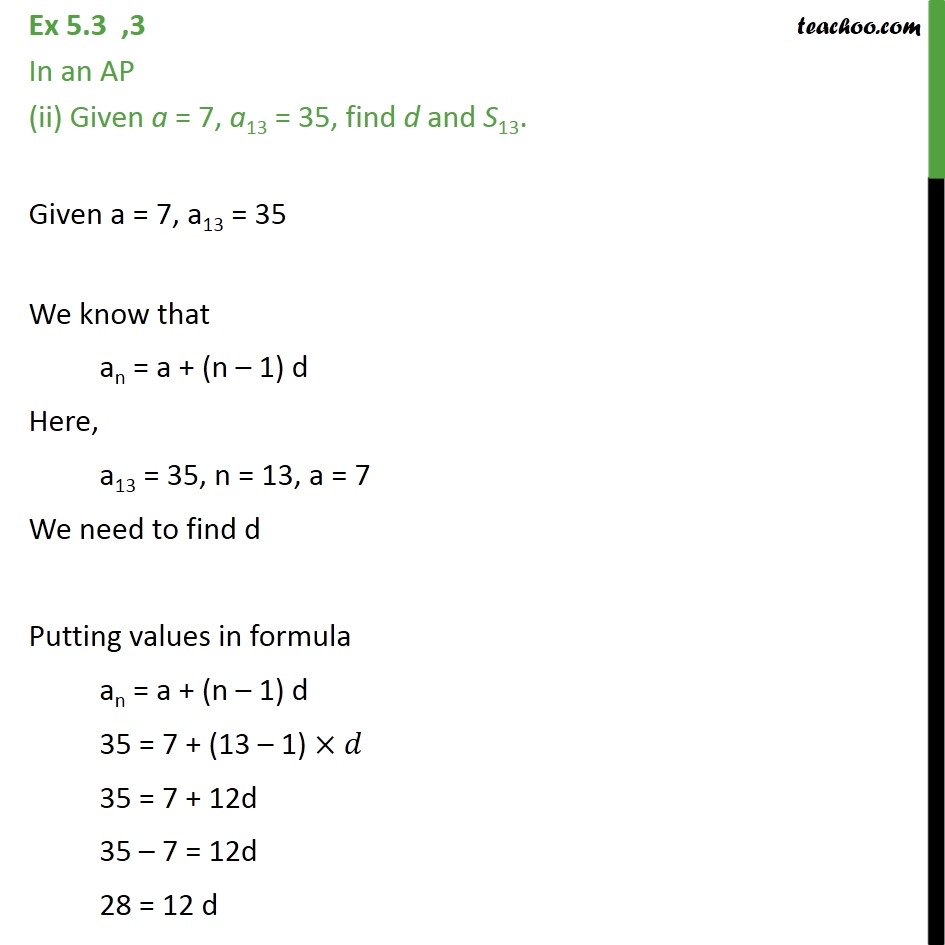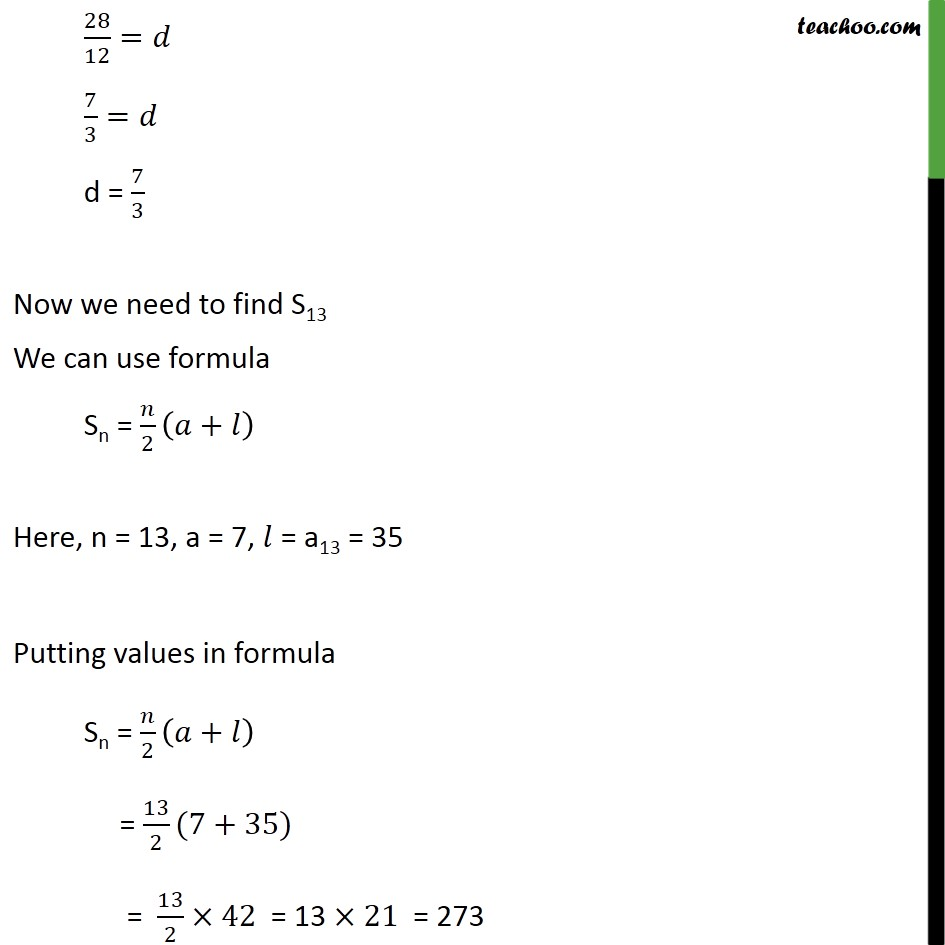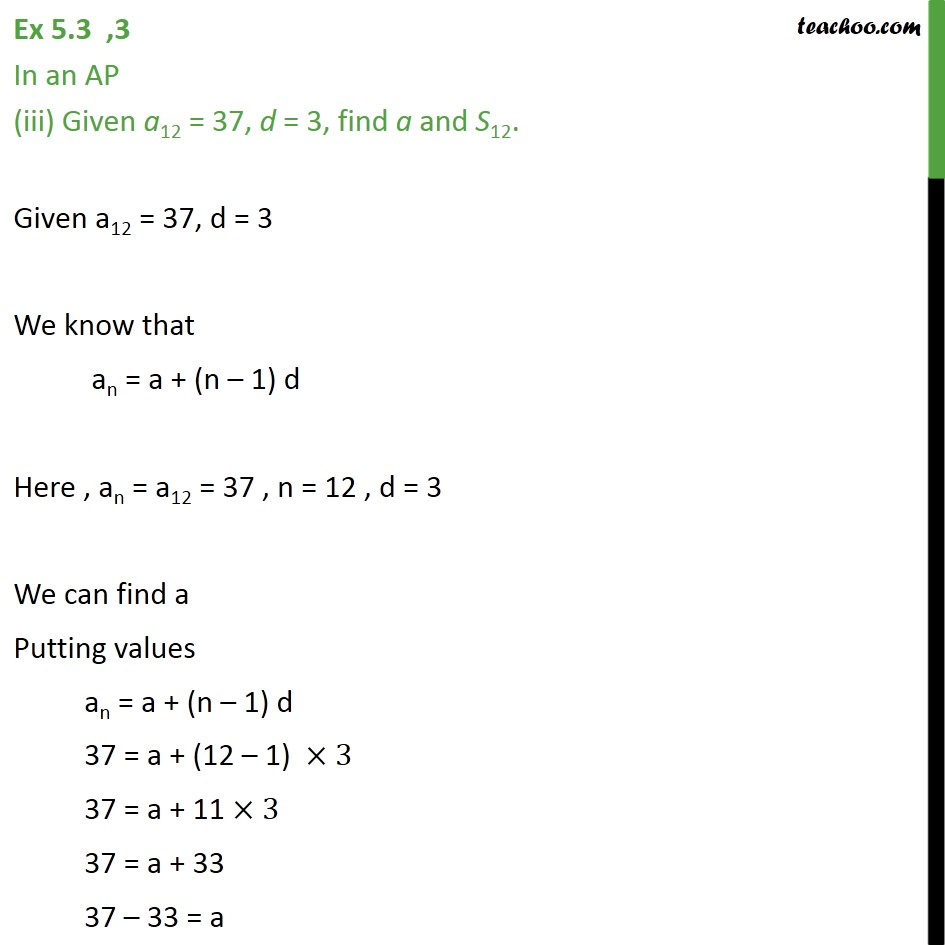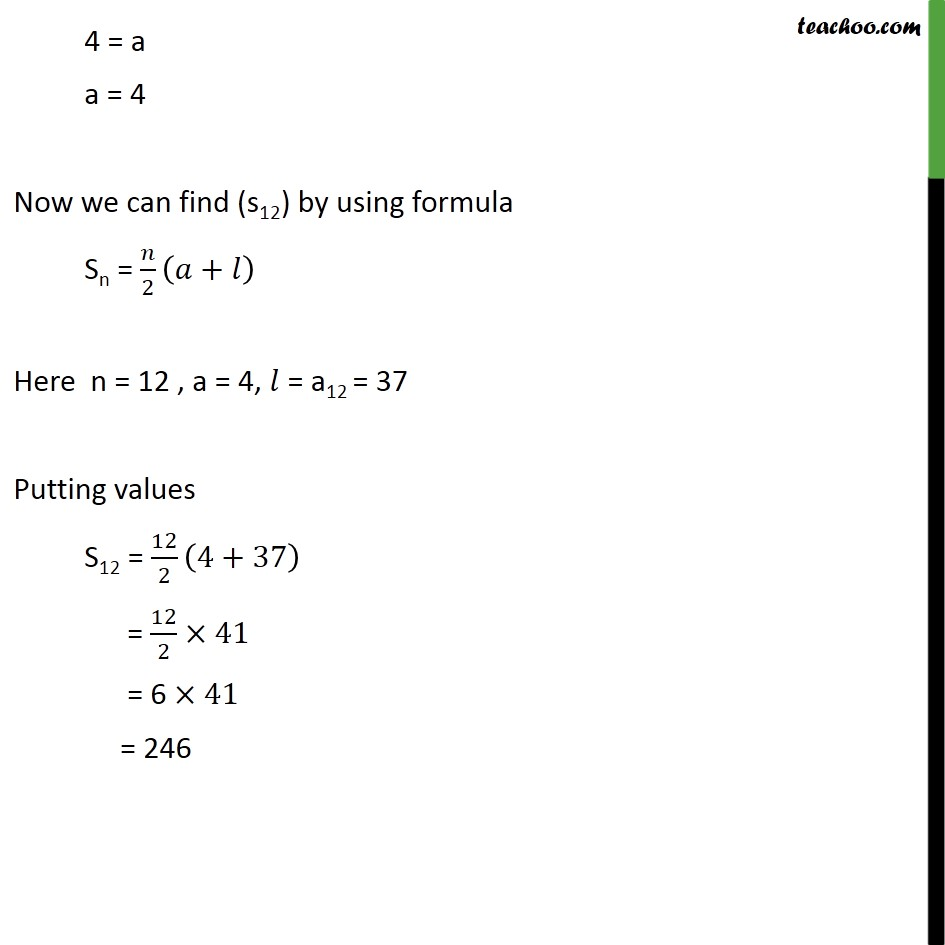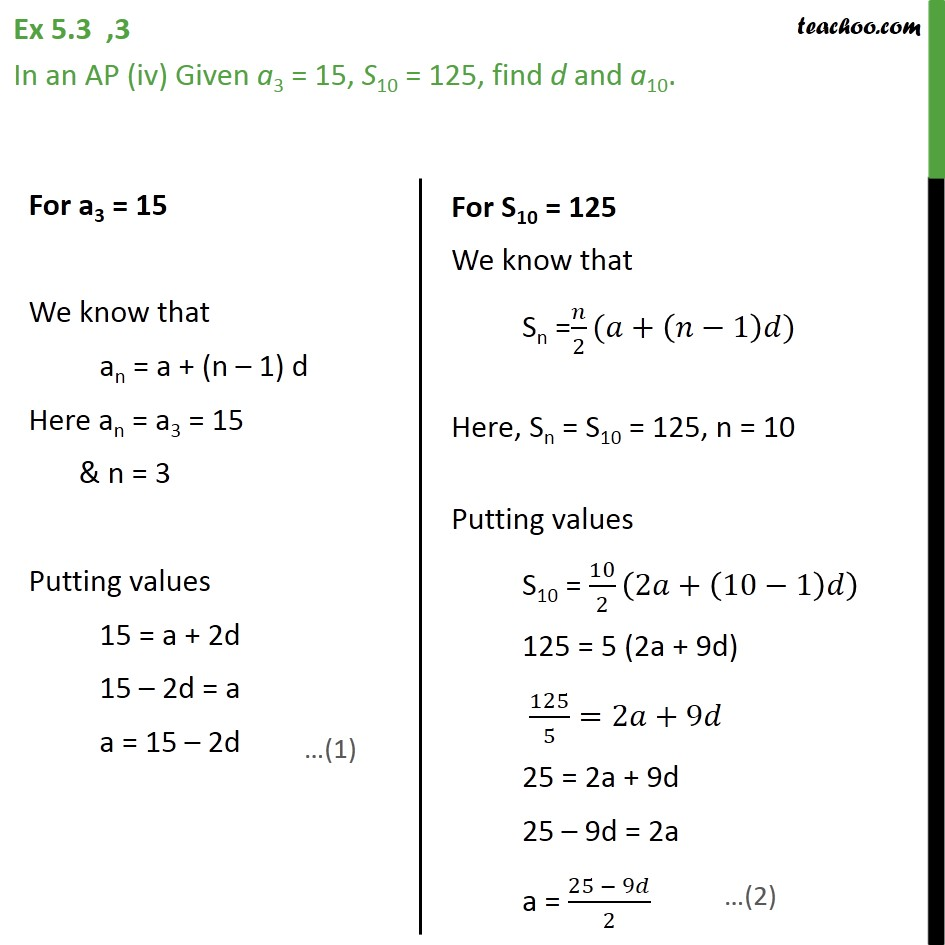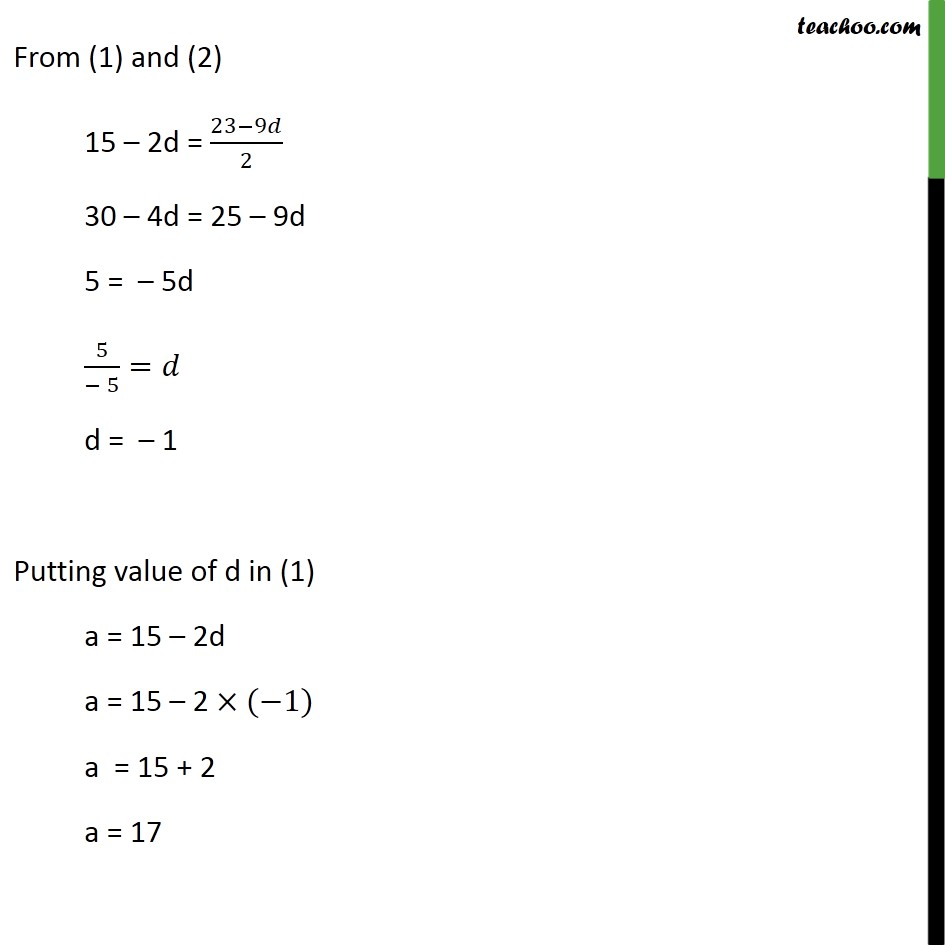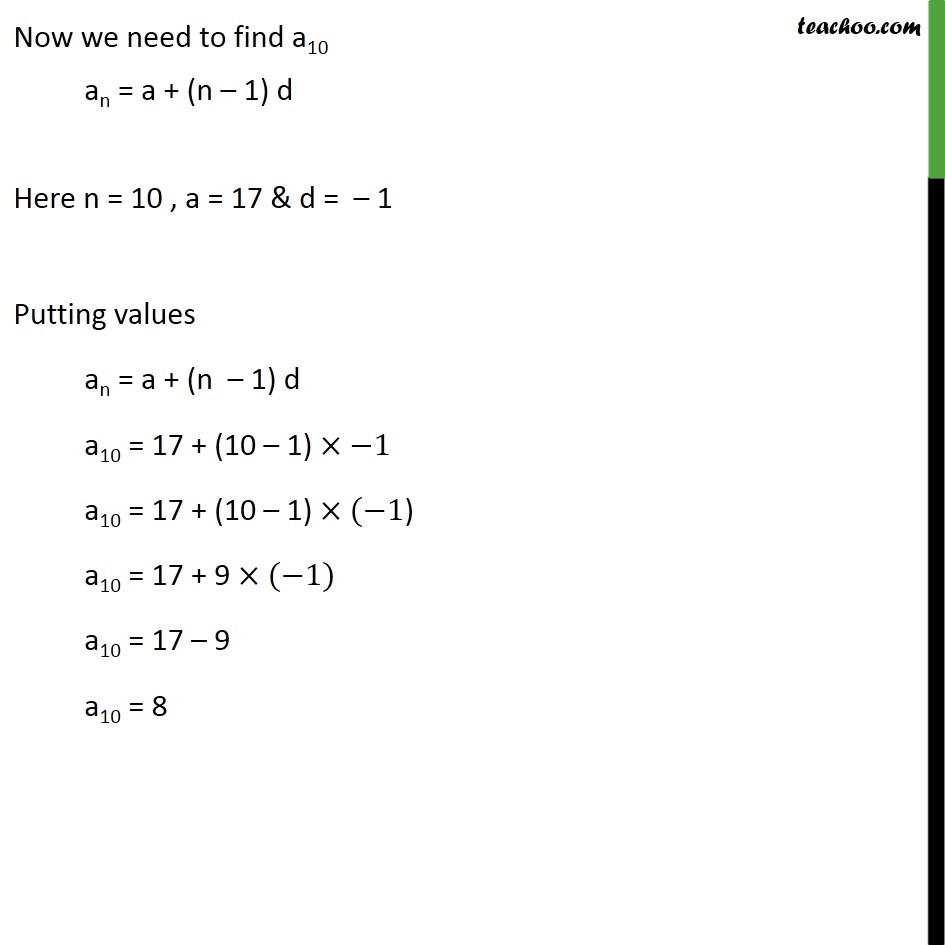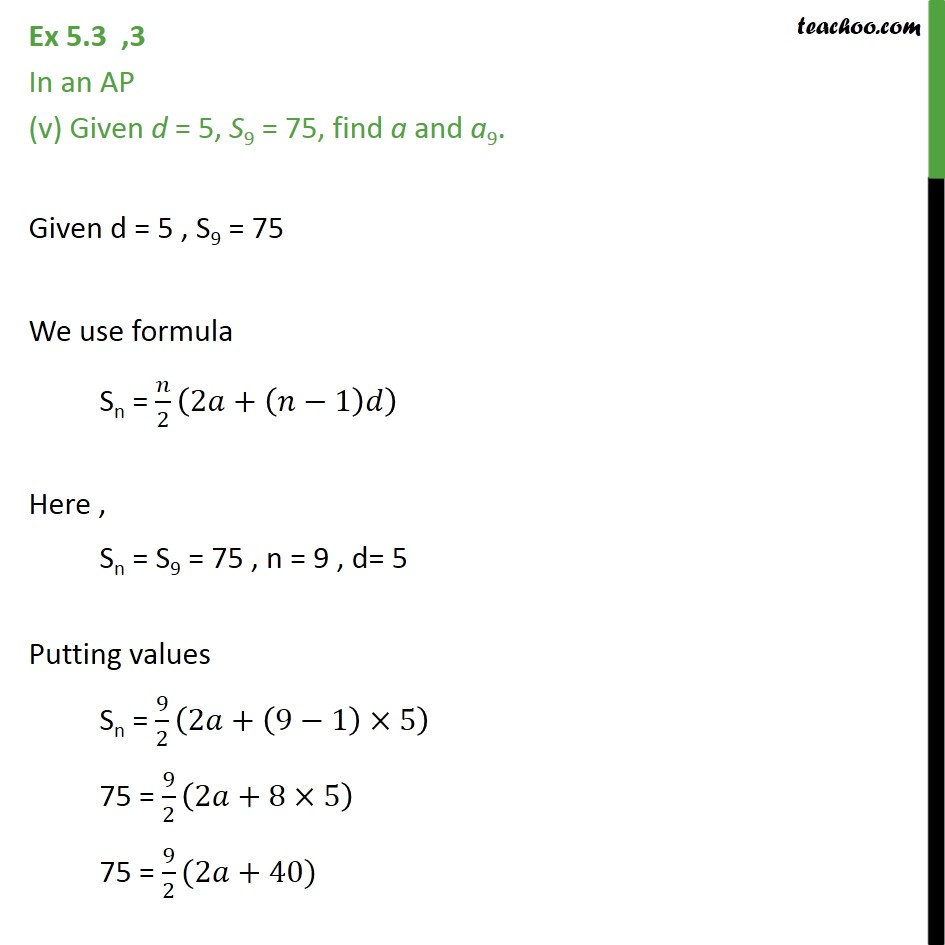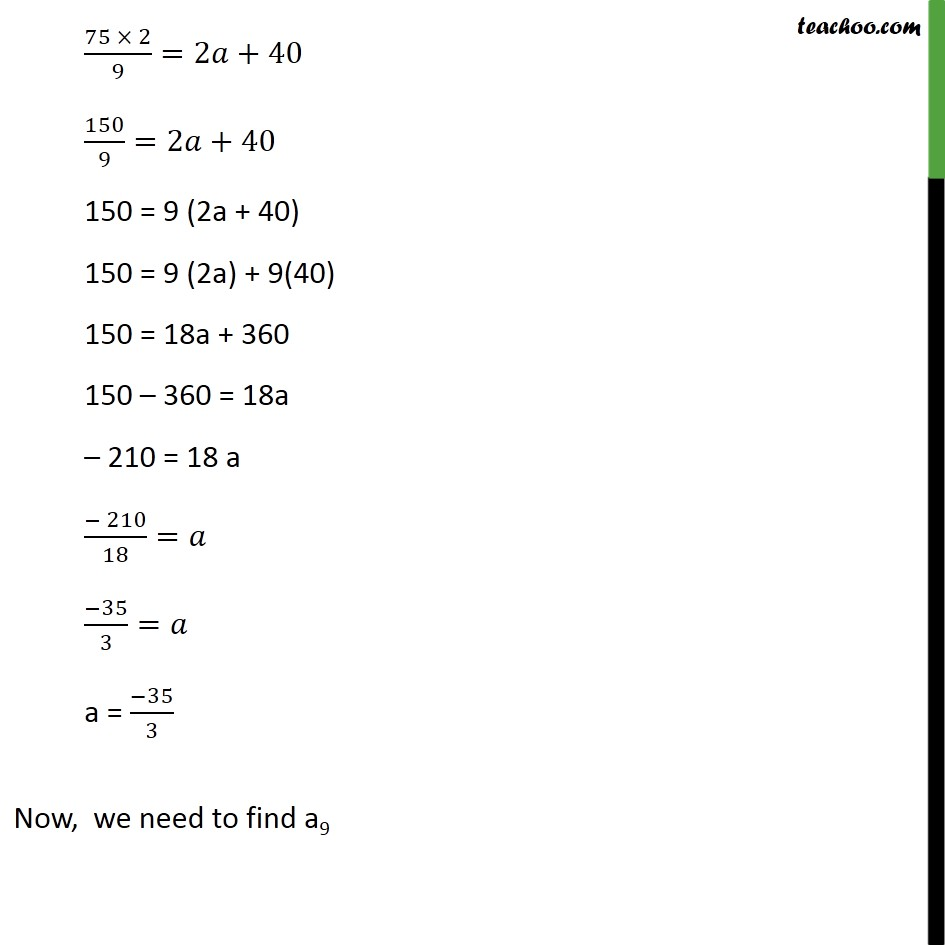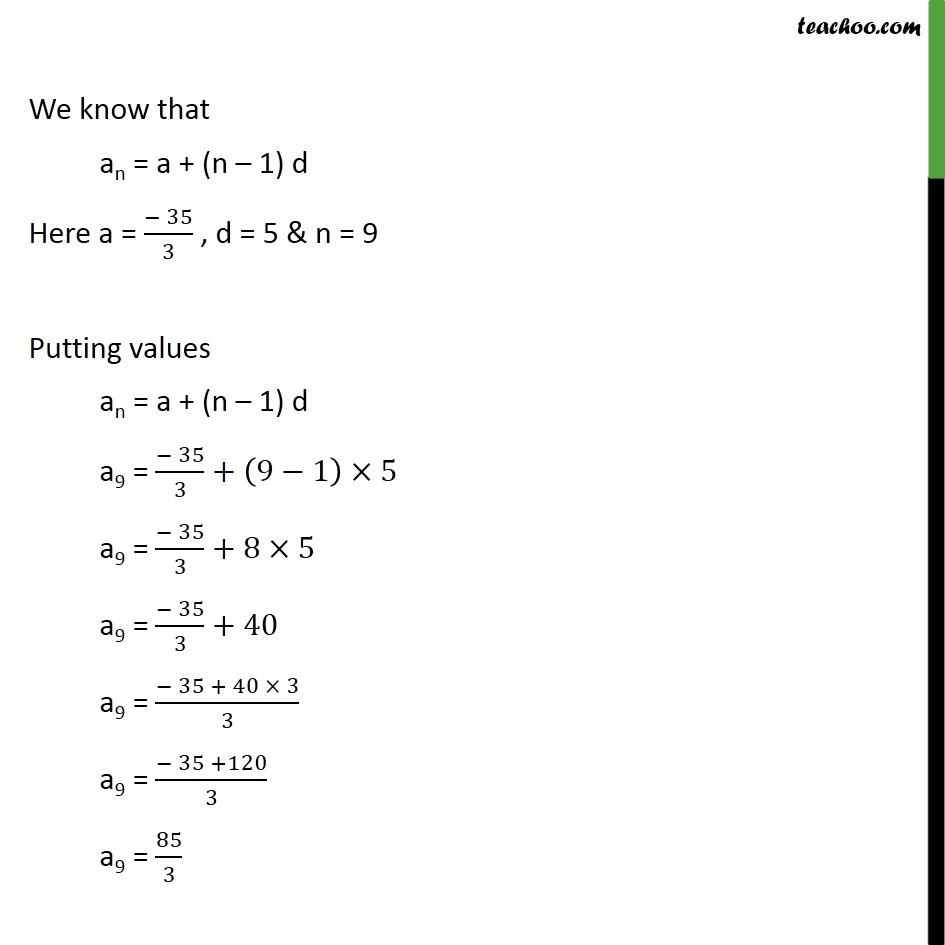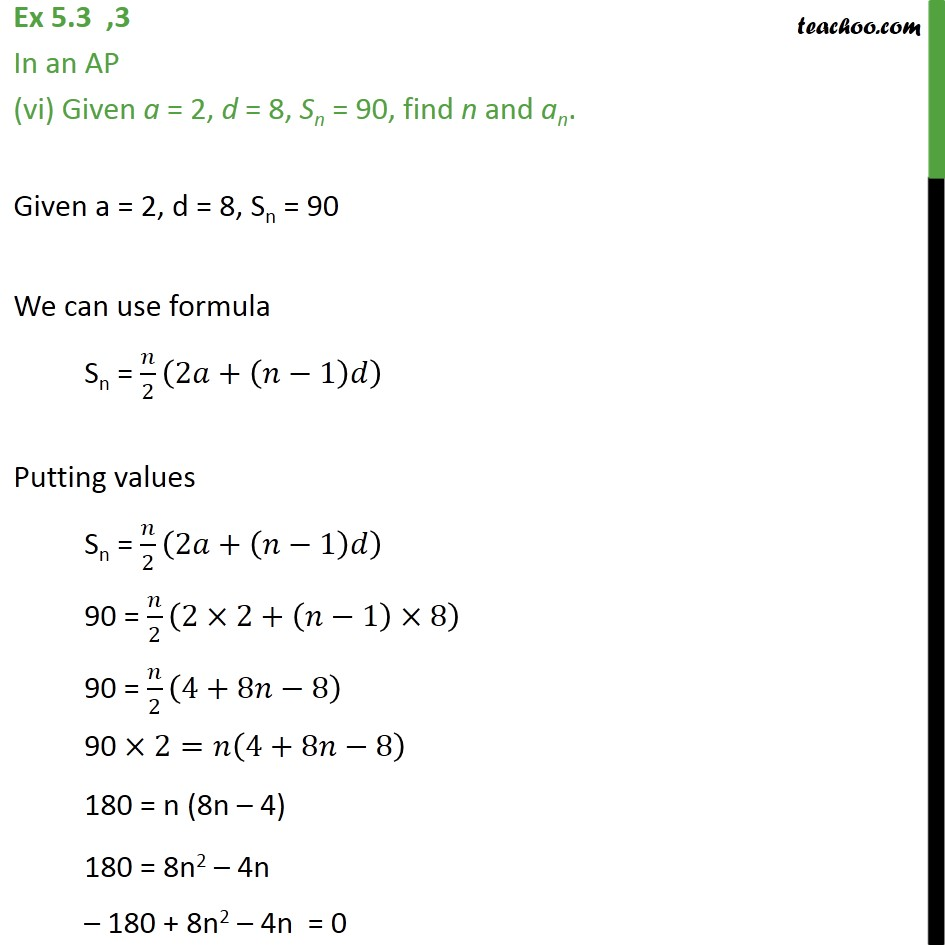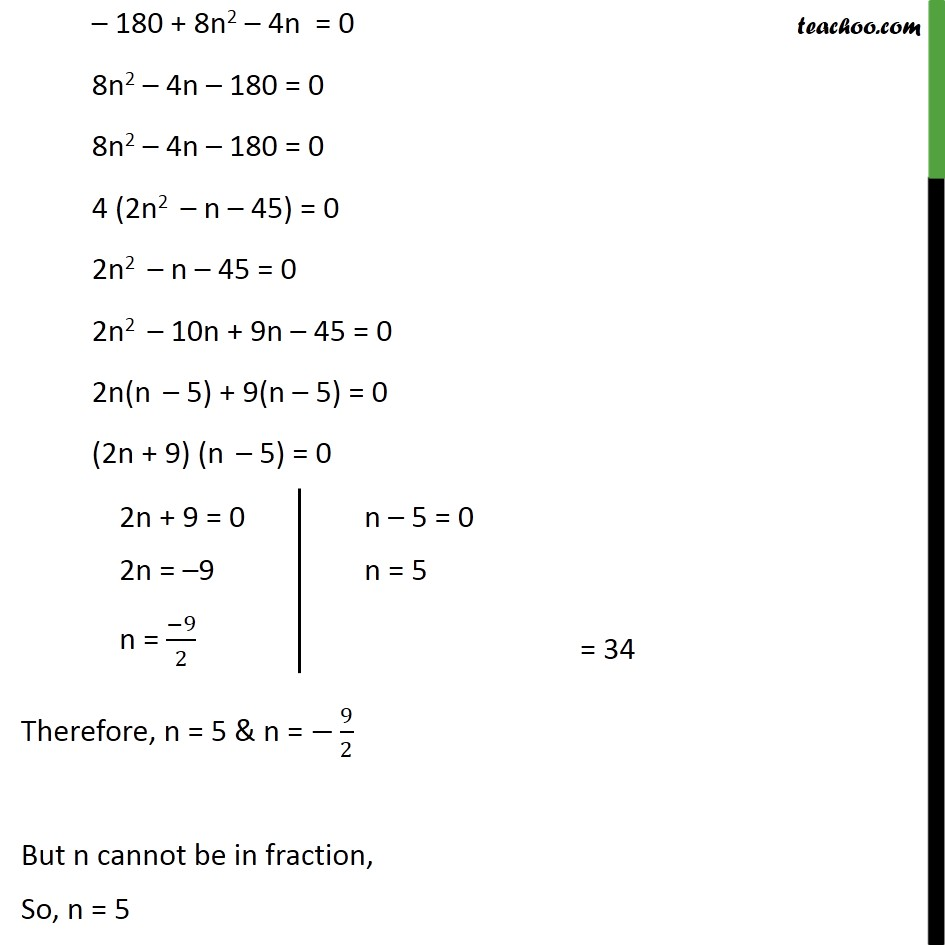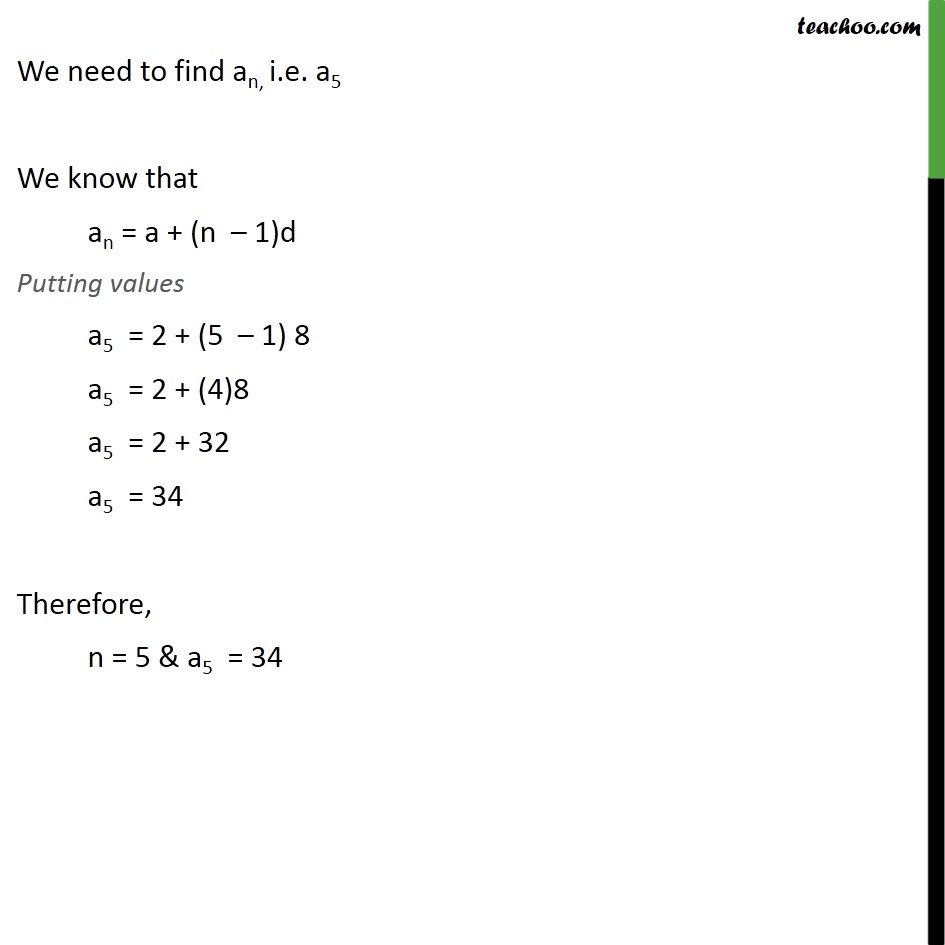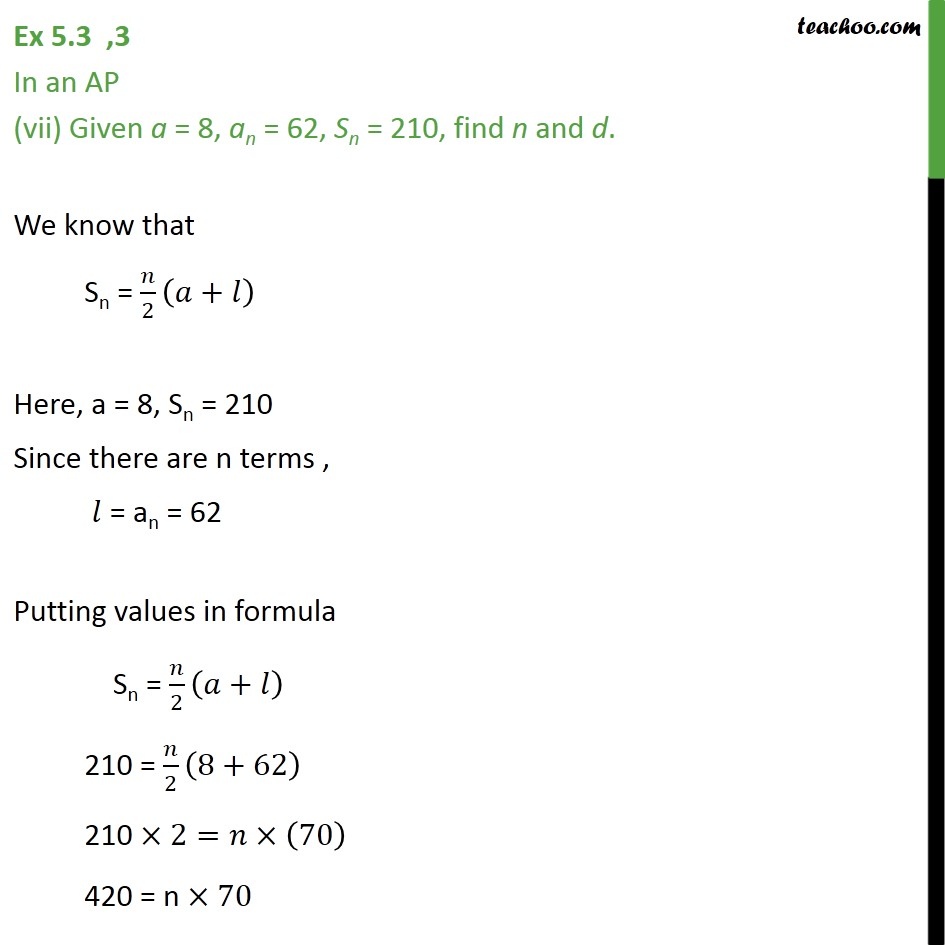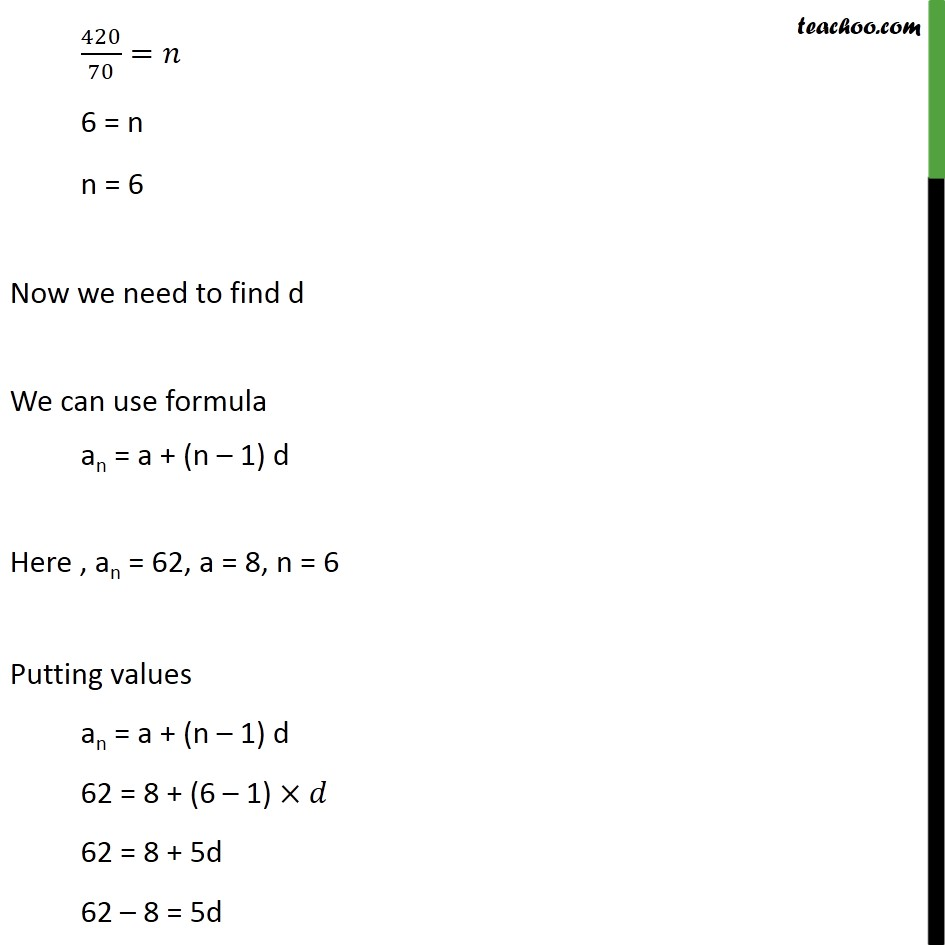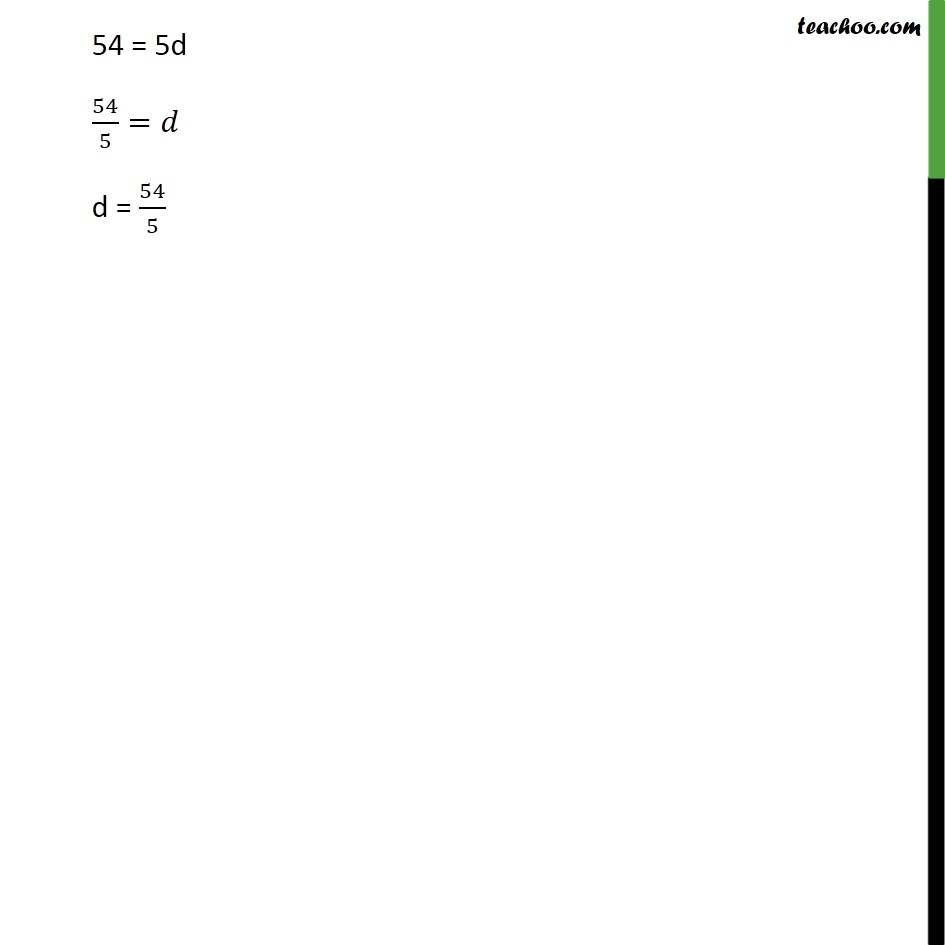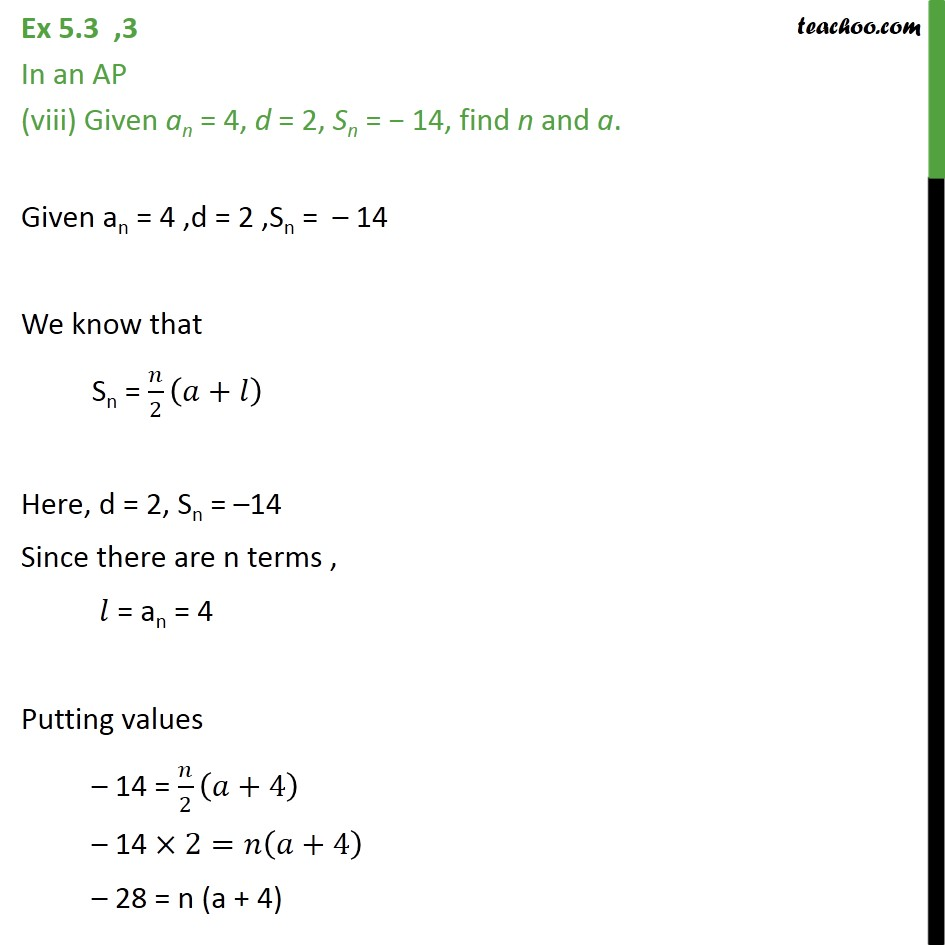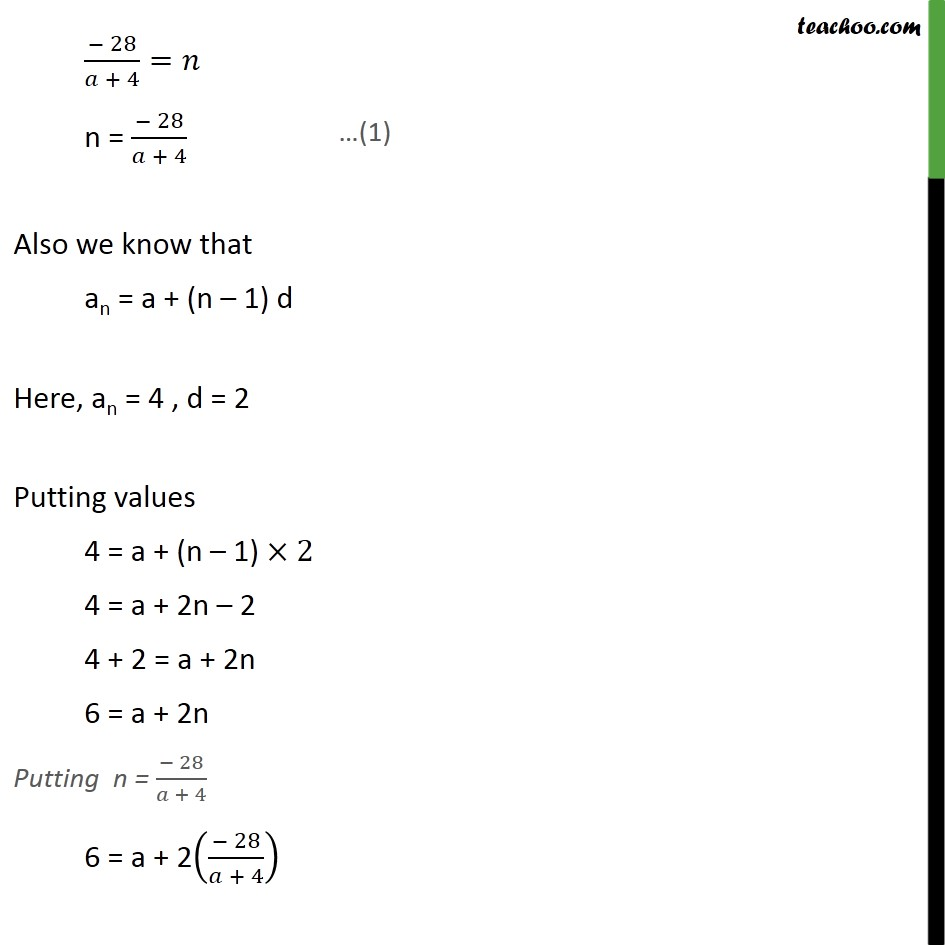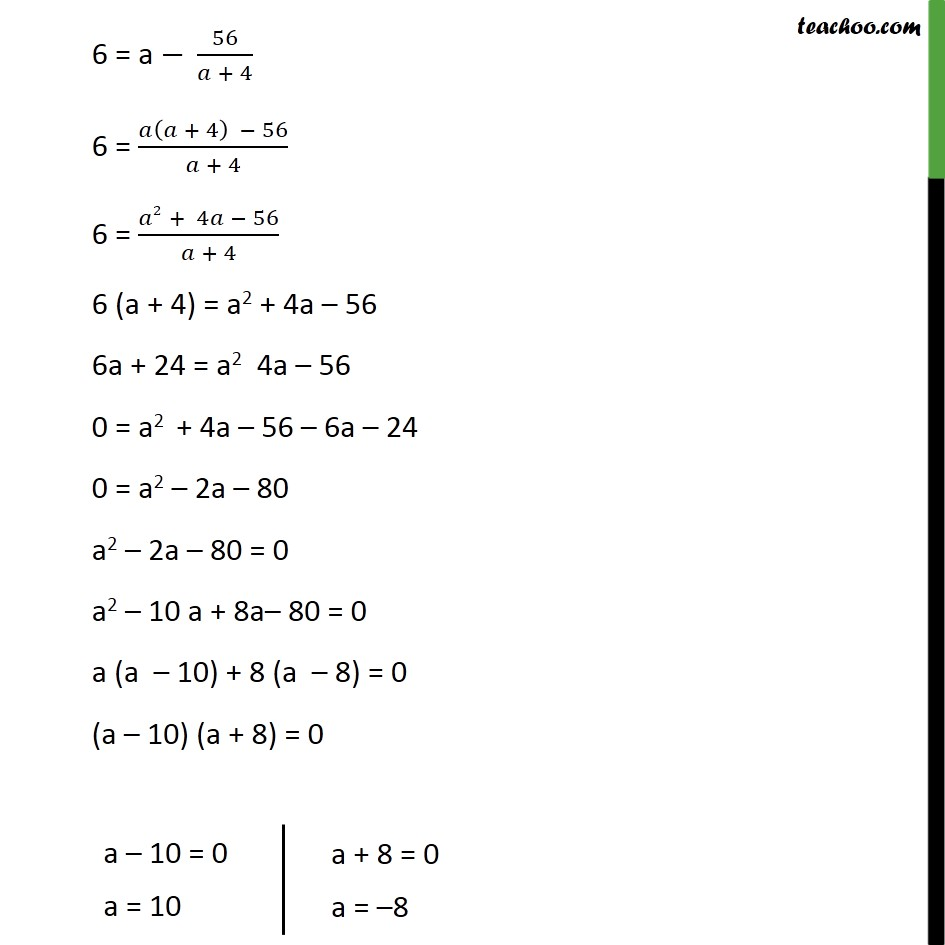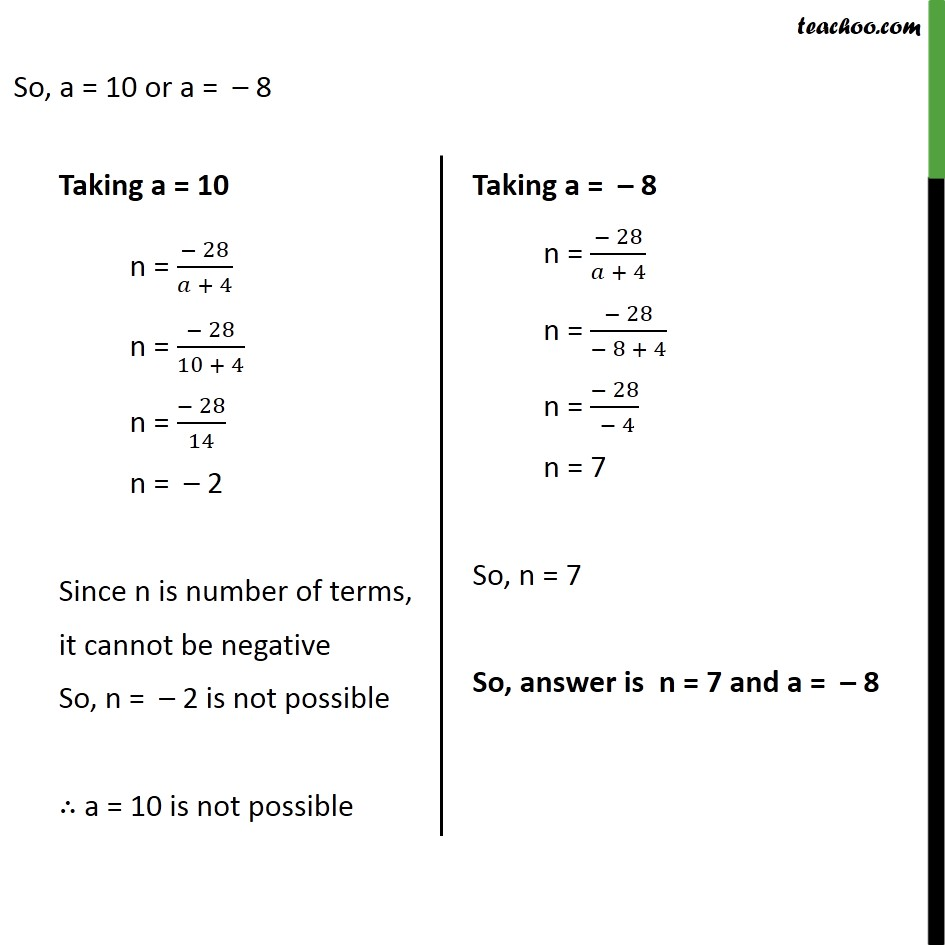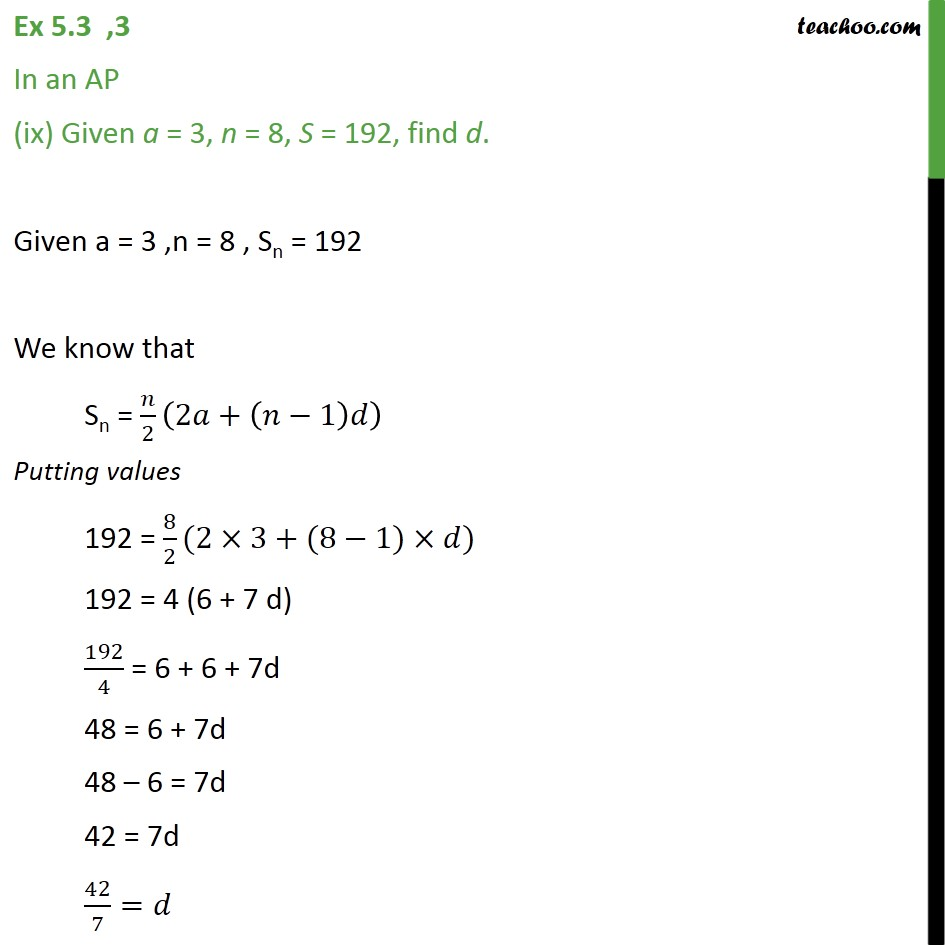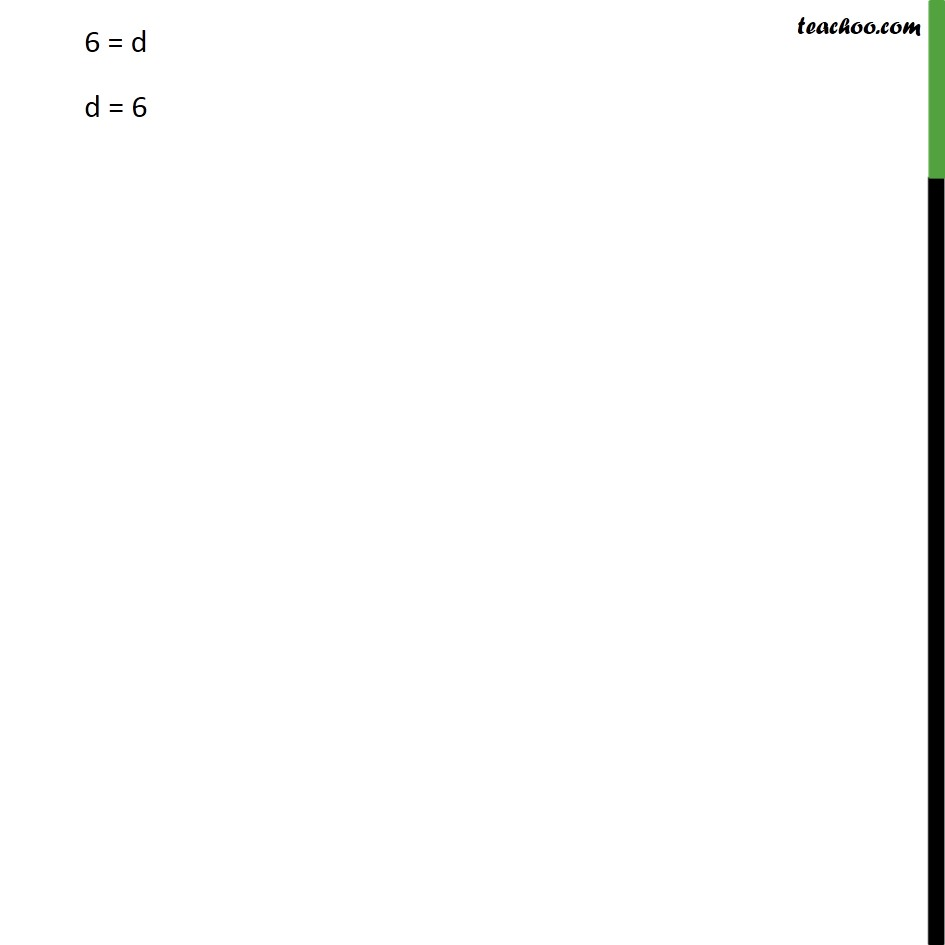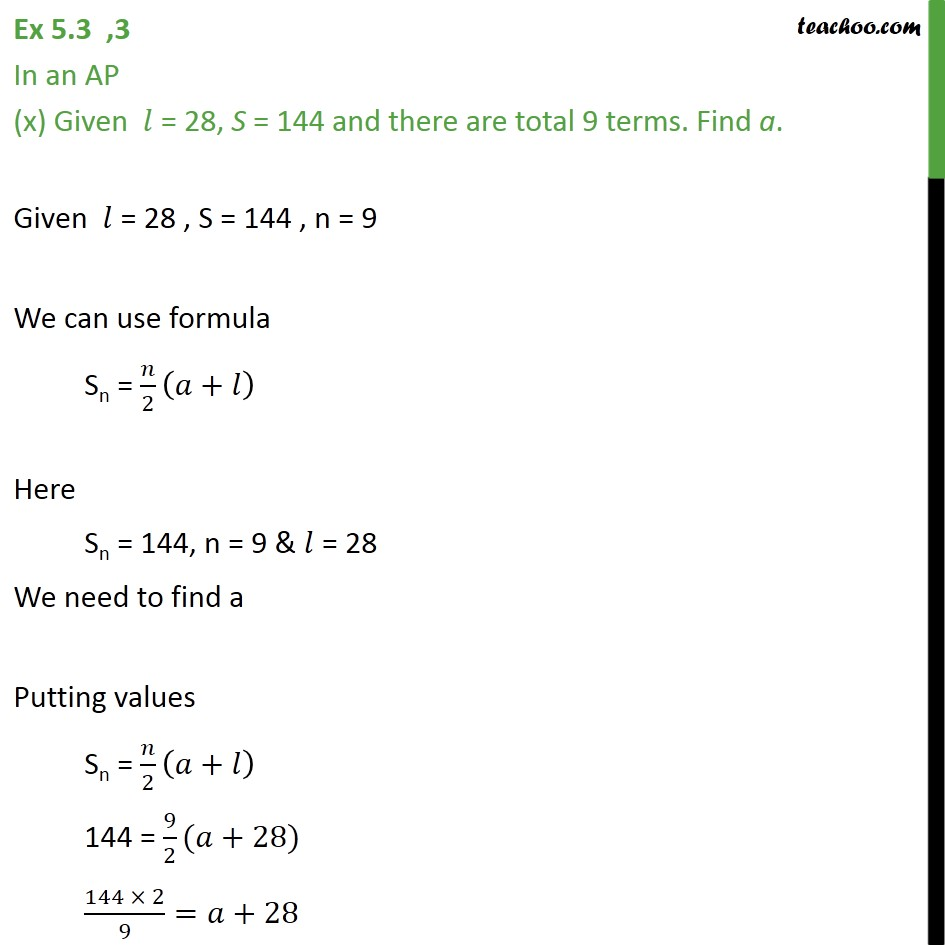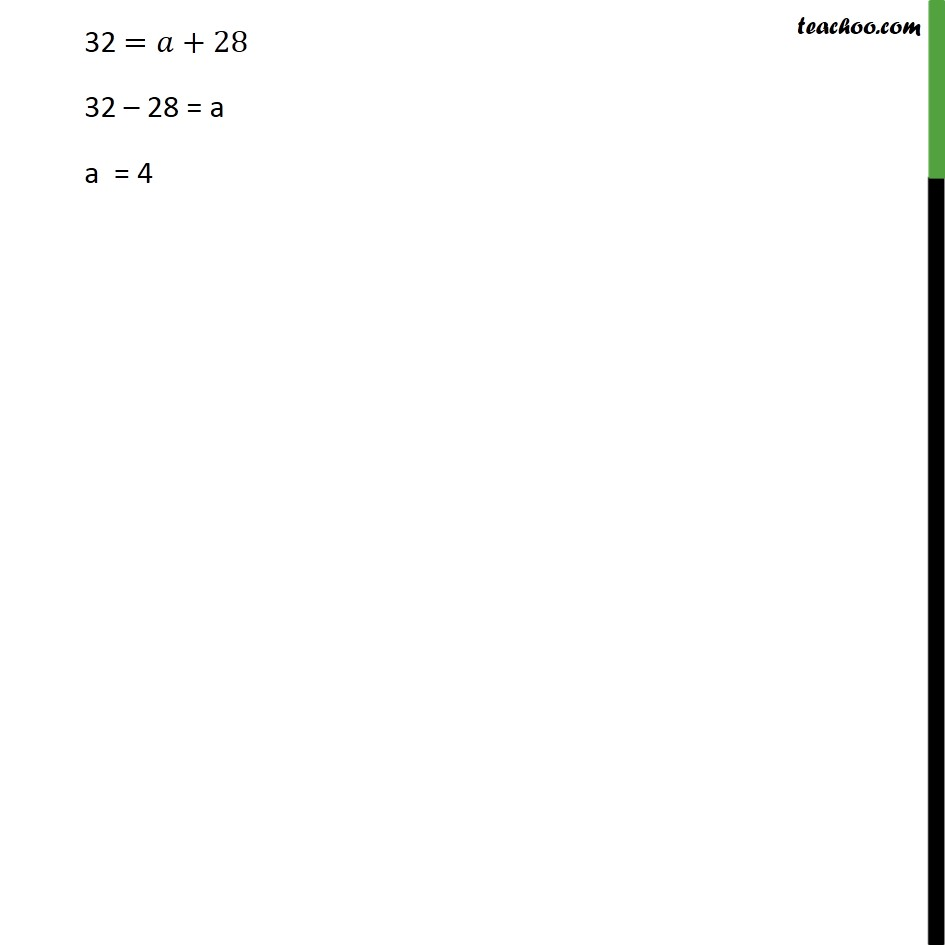1. Chapter 5 Class 10 Arithmetic Progressions
2. Serial order wise
3. Ex 5.3

Transcript

Ex 5.3 ,3 In an AP (i) Given a = 5, d = 3, an = 50, find n and Sn. Given a = 5 , d = 3 , an = 50 We know that an = a + (n 1) d Putting values 50 = 5 + (n 1) 3 50 = 5 + 3n 3 50 = 2 + 3n 50 2 = 3n 48 = 3n 48/3= n = 16 Now we need to find Sn Sn = /2(2 +( 1) Putting n = 16, a = 5, d = 3 Sn = /2 (2 +( 1) ) = 16/2 (2 5+(16 1) 3) = 8 (10+15 3) = 8(10+45) = 8 55 = 440 Ex 5.3 ,3 In an AP (ii) Given a = 7, a13 = 35, find d and S13. Given a = 7, a13 = 35 We know that an = a + (n 1) d Here, a13 = 35, n = 13, a = 7 We need to find d Putting values in formula an = a + (n 1) d 35 = 7 + (13 1) 35 = 7 + 12d 35 7 = 12d 28 = 12 d 28/12= 7/3= d = 7/3 Now we need to find S13 We can use formula Sn = /2 ( + ) Here, n = 13, a = 7, = a13 = 35 Putting values in formula Sn = /2 ( + ) = 13/2(7+35) = 13/2 42 = 13 21 = 273 Ex 5.3 ,3 In an AP (iii) Given a12 = 37, d = 3, find a and S12. Given a12 = 37, d = 3 We know that an = a + (n 1) d Here , an = a12 = 37 , n = 12 , d = 3 We can find a Putting values an = a + (n 1) d 37 = a + (12 1) 3 37 = a + 11 3 37 = a + 33 37 33 = a 4 = a a = 4 Now we can find (s12) by using formula Sn = /2 ( + ) Here n = 12 , a = 4, = a12 = 37 Putting values S12 = 12/2 (4+37) = 12/2 41 = 6 41 = 246 Ex 5.3 ,3 In an AP (iv) Given a3 = 15, S10 = 125, find d and a10. From (1) and (2) 15 2d = (23 9 )/2 30 4d = 25 9d 5 = 5d 5/( 5)= d = 1 Putting value of d in (1) a = 15 2d a = 15 2 ( 1) a = 15 + 2 a = 17 Now we need to find a10 an = a + (n 1) d Here n = 10 , a = 17 & d = 1 Putting values an = a + (n 1) d a10 = 17 + (10 1) 1 a10 = 17 + (10 1) ( 1) a10 = 17 + 9 ( 1) a10 = 17 9 a10 = 8 Ex 5.3 ,3 In an AP (v) Given d = 5, S9 = 75, find a and a9. Given d = 5 , S9 = 75 We use formula Sn = /2 (2 +( 1) ) Here , Sn = S9 = 75 , n = 9 , d= 5 Putting values Sn = 9/2 (2 +(9 1) 5) 75 = 9/2 (2 +8 5) 75 = 9/2(2 +40) (75 2)/9=2 +40 150/9=2 +40 150 = 9 (2a + 40) 150 = 9 (2a) + 9(40) 150 = 18a + 360 150 360 = 18a 210 = 18 a ( 210)/18= ( 35)/3= a = ( 35)/3 Now, we need to find a9 We know that an = a + (n 1) d Here a = ( 35)/3 , d = 5 & n = 9 Putting values an = a + (n 1) d a9 = ( 35)/3+(9 1) 5 a9 = ( 35)/3+8 5 a9 = ( 35)/3+40 a9 = ( 35 + 40 3)/3 a9 = ( 35 +120)/3 a9 = 85/3 Ex 5.3 ,3 In an AP (vi) Given a = 2, d = 8, Sn = 90, find n and an. Given a = 2, d = 8, Sn = 90 We can use formula Sn = /2 (2 +( 1) ) Putting values Sn = /2 (2 +( 1) ) 90 = /2 (2 2+( 1) 8) 90 = /2 (4+8 8) 90 2= (4+8 8) 180 = n (8n 4) 180 = 8n2 4n 180 + 8n2 4n = 0 180 + 8n2 4n = 0 8n2 4n 180 = 0 8n2 4n 180 = 0 4 (2n2 n 45) = 0 2n2 n 45 = 0 2n2 10n + 9n 45 = 0 2n(n 5) + 9(n 5) = 0 (2n + 9) (n 5) = 0 We need to find an, i.e. a5 We know that an = a + (n – 1)d Putting values a5 = 2 + (5 – 1) 8 a5 = 2 + (4)8 a5 = 2 + 32 a5 = 34 Therefore, n = 5 & a5 = 34 Ex 5.3 ,3 In an AP (vii) Given a = 8, an = 62, Sn = 210, find n and d. We know that Sn = /2 ( + ) Here, a = 8, Sn = 210 Since there are n terms , = an = 62 Putting values in formula Sn = /2 ( + ) 210 = /2 (8+62) 210 2= (70) 420 = n 70 420/70= 6 = n n = 6 Now we need to find d We can use formula an = a + (n 1) d Here , an = 62, a = 8, n = 6 Putting values an = a + (n 1) d 62 = 8 + (6 1) 62 = 8 + 5d 62 8 = 5d 54 = 5d 54/5= d = 54/5 Ex 5.3 ,3 In an AP (viii) Given an = 4, d = 2, Sn = 14, find n and a. Given an = 4 ,d = 2 ,Sn = 14 We know that Sn = /2 ( + ) Here, d = 2, Sn = 14 Since there are n terms , = an = 4 Putting values 14 = /2 ( +4) 14 2= ( +4) 28 = n (a + 4) ( 28)/( + 4)= n = ( 28)/( + 4) Also we know that an = a + (n 1) d Here, an = 4 , d = 2 Putting values 4 = a + (n 1) 2 4 = a + 2n 2 4 + 2 = a + 2n 6 = a + 2n Putting n = ( 28)/( + 4) 6 = a + 2(( 28)/( + 4)) 6 = a 56/( + 4) 6 = ( ( + 4) 56)/( + 4) 6 = ( 2 + 4 56)/( + 4) 6 (a + 4) = a2 + 4a 56 6a + 24 = a2 4a 56 0 = a2 + 4a 56 6a 24 0 = a2 2a 80 a2 2a 80 = 0 a2 10 a + 8a 80 = 0 a (a 10) + 8 (a 8) = 0 (a 10) (a + 8) = 0 So, a = 10 or a = 8 Ex 5.3 ,3 In an AP (ix) Given a = 3, n = 8, S = 192, find d. Given a = 3 ,n = 8 , Sn = 192 We know that Sn = /2 (2 +( 1) ) Putting values 192 = 8/2(2 3+(8 1) ) 192 = 4 (6 + 7 d) 192/4 = 6 + 6 + 7d 48 = 6 + 7d 48 6 = 7d 42 = 7d 42/7= 6 = d d = 6 Ex 5.3 ,3 In an AP (x) Given = 28, S = 144 and there are total 9 terms. Find a. Given = 28 , S = 144 , n = 9 We can use formula Sn = /2 ( + ) Here Sn = 144, n = 9 & = 28 We need to find a Putting values Sn = /2 ( + ) 144 = 9/2( +28) (144 2)/9= +28 32 = +28 32 28 = a a = 4

Ex 5.3